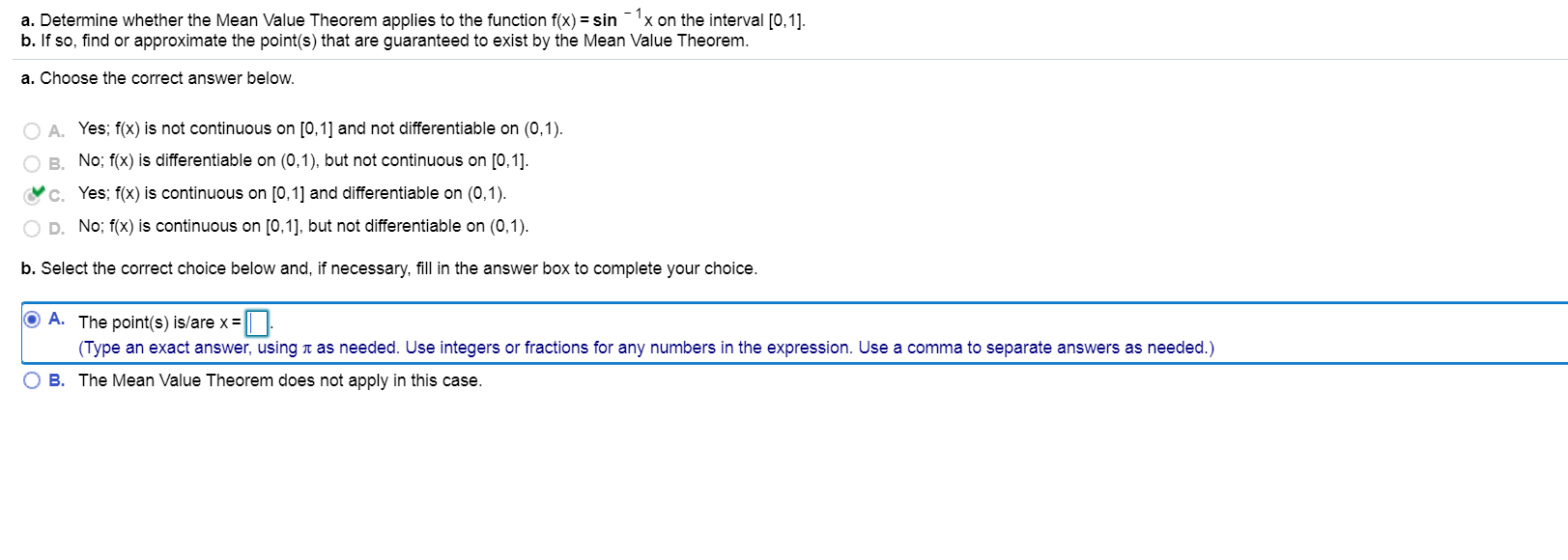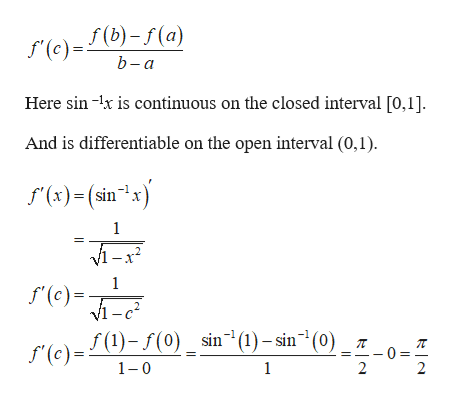# a. Determine whether the Mean Value Theorem applies to the function f(x)= sinx on the interval [0,1]b. If so, find or approximate the point(s) that are guaranteed to exist by the Mean Value Theorema. Choose the correct answer below.A. Yes; f(x) is not continuous on [0,1] and not differentiable on (0,1)B. No; f(x) is differentiable on (0,1), but not continuous on [0,1].c. Yes; f(x) is continuous on [0,1] and differentiable on (0,1)OD.No; f(x) is continuous on [0,1], but not differentiable on (0,1)b. Select the correct choice below and, if necessary, fill in the answer box to complete your choice.O A. The point(s) is/are x=(Type an exact answer, using t as needed. Use integers or fractions for any numbers in the expression. Use a comma to separate answers as needed.)B. The Mean Value Theorem does not apply in this case.

Question
2 viewshelp_outlineImage Transcriptionclosea. Determine whether the Mean Value Theorem applies to the function f(x)= sinx on the interval [0,1] b. If so, find or approximate the point(s) that are guaranteed to exist by the Mean Value Theorem a. Choose the correct answer below. A. Yes; f(x) is not continuous on [0,1] and not differentiable on (0,1) B. No; f(x) is differentiable on (0,1), but not continuous on [0,1]. c. Yes; f(x) is continuous on [0,1] and differentiable on (0,1) OD.No; f(x) is continuous on [0,1], but not differentiable on (0,1) b. Select the correct choice below and, if necessary, fill in the answer box to complete your choice. O A. The point(s) is/are x= (Type an exact answer, using t as needed. Use integers or fractions for any numbers in the expression. Use a comma to separate answers as needed.) B. The Mean Value Theorem does not apply in this case. fullscreen
check_circle

star
star
star
star
star
1 Rating
Step 1

Mean value theorem:

If f(x) is a function that satisfies both of the following rules.

(i) f(x) is continuous on the closed interval [a, b].

(ii) f(x) is...help_outlineImage Transcriptionclosef (b)-f(a) f(c) b-а Here sin -x is continuous on the closed interval [0,1]. And is differentiable on the open interval (0,1) f(x)(sinx 1 М-х? 1 f(c) vI-c2 f (1)-(0) sin(1)-sin"(0) (e) 1 0 1 2 2 fullscreen

### Want to see the full answer?

See Solution

#### Want to see this answer and more?

Solutions are written by subject experts who are available 24/7. Questions are typically answered within 1 hour.*

See Solution
*Response times may vary by subject and question.
Tagged in

### Derivative